# Accurately measure resistance with less-than-perfect components

For transducers, such as strain gauges or thermistors, you must accurately and inexpensively measure resistance using circuitry built with imperfect components and in which gain and offset errors can significantly limit the accuracy of ohmic measurements. The right circuit topology makes it possible to eliminate most error terms while measuring ohms, leaving the accuracy to be determined by just a single reference resistor (Figure 1).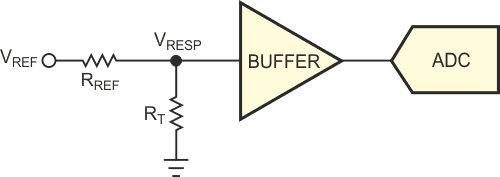Figure 1. The resistive-divider topology provides a lower cost alternative to a current source and a precision resistor for calibration.

Unlike measuring voltage or current, measuring a passive attribute, such as resistance, requires a stimulus. One method of measuring resistance is to force a known current through a resistor and measure the voltage across the resistor. Measuring ohms in this way means that, with the correct selection of stimulus current, you need do no math, so this method was popular when the cost of computation was more than the cost of building an accurate current source. However, the accuracy of the current source directly limits the accuracy of the reading and any gain or offset errors from measuring the response voltage offsets the accuracy, as well. Additionally, the range of measurement is limited to the ADC's signal range, as the following equation shows: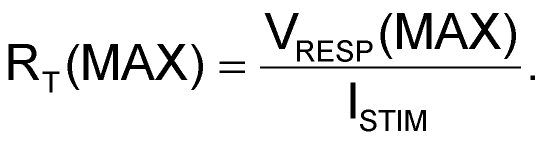With the development of more powerful microcontrollers and on-chip ratiometric ADCs, a resistor-divider block architecture (Figure 2) provides a less expensive approach: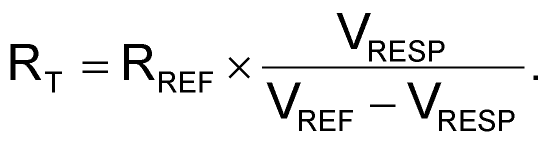This architecture has a theoretical range of measurement from short circuit to open circuit, but any offset error from measuring the response voltage limits the actual range; the reference resistance limits overall accuracy and any gain and offset errors from measuring the response voltage.

The cost of the reference resistor determines the error that the reference resistor introduces, and you derive the supply voltage, VCC, from the reference voltage, VREF. The gain error of a ratiometric ADC is generally small and does not contribute much to the overall error, but this situation is not the case for the offset error, which can be the largest contributor of error to the overall accuracy. Using more expensive and precise components reduces the offset error of any op amps in the measurement path.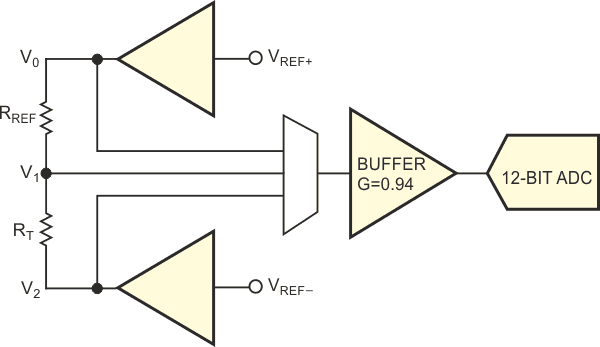Figure 2. Remove most gain and offset errors using two measurements and a ratio calculation.

Figure 2 shows how to significantly remove gain and offset errors, in which subtracting two measured voltages removes any offset errors in the measurement system: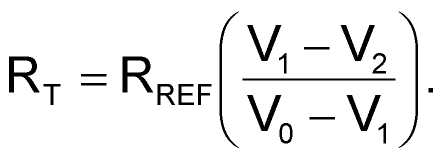The ratio of these two difference values removes any measurement-path gain error, leaving the reference resistance to determine the measurement error. This result is valid as long as the measured signal is never outside the range of the A/D converter. To guarantee this condition, set the sense buffer gain to slightly less than unity.

You can also measure multiple resistors, in which all the sense paths multiplex to a single buffer and A/D converter, and the eight analog pins let you measure as many as six transducers (Figure 3). Alternatively, you could connect each of four sense paths to its own buffer and converter.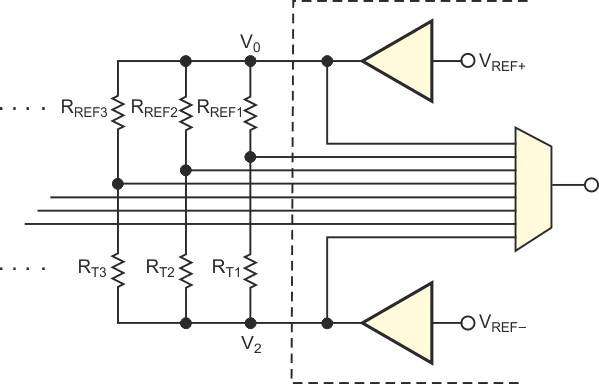Figure 3. Extend the idea to handle multiple sensors and signal paths, using multiplexing through a single buffer and A/D converter.

The listing, which can be downloaded from the link at the end of the article, shows how you implement the circuit of Figure 2 using a programmable analog system-on-chip controller. It uses the Cypress’ ADCINC12 user module (Ref. 1), programmable-gain-adjustment user module, and two analog output buffers. Placing the analog block of the ADCINC12 just below the buffer and setting the clock for the ADCINC12 to 167 kHz for a sample rate of 10 samples/sec remove any 50- or 60-Hz interference from the signal. Increase the sample rate if the application requires a faster conversion. The control software is in C; the program calculates the resistance reading and leaves it in a global memory location.